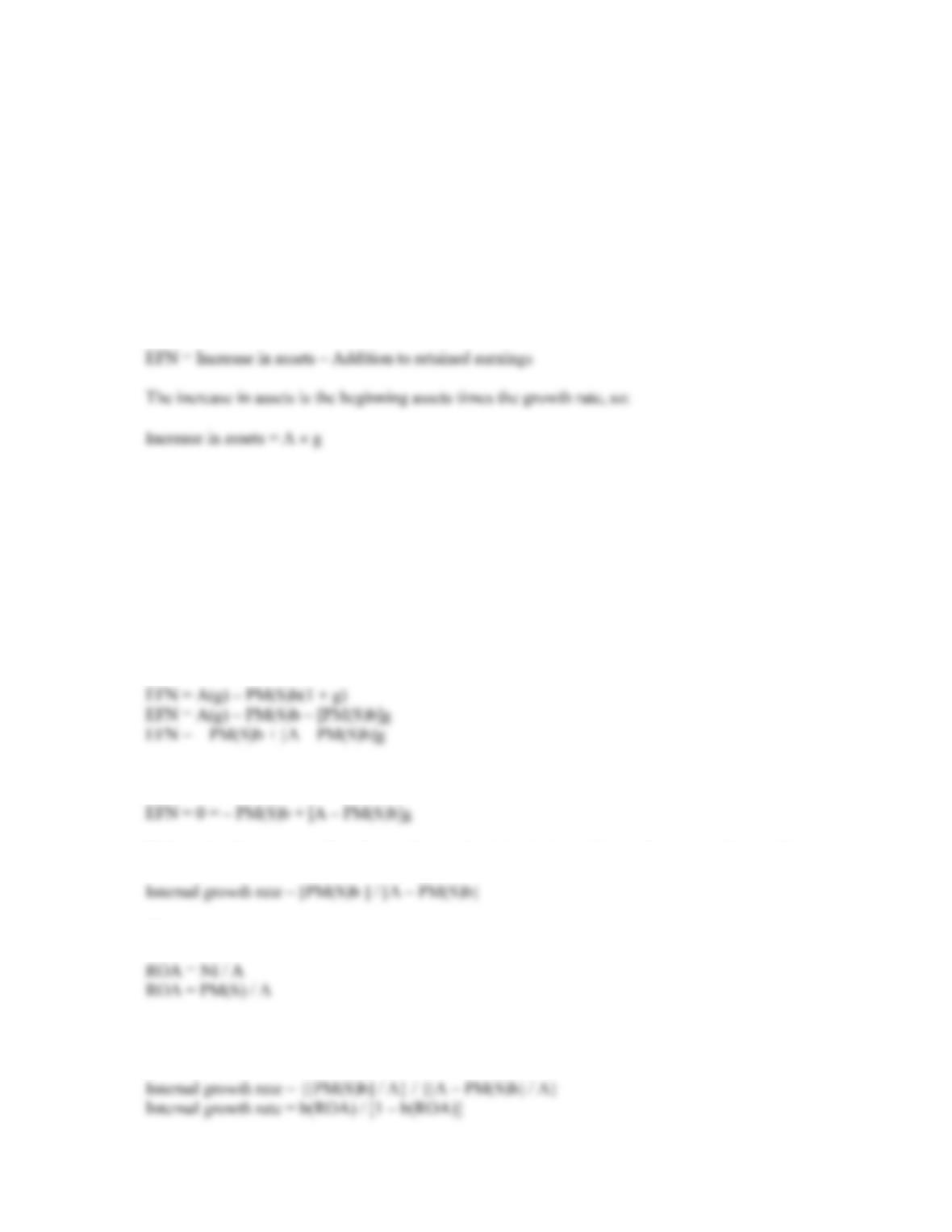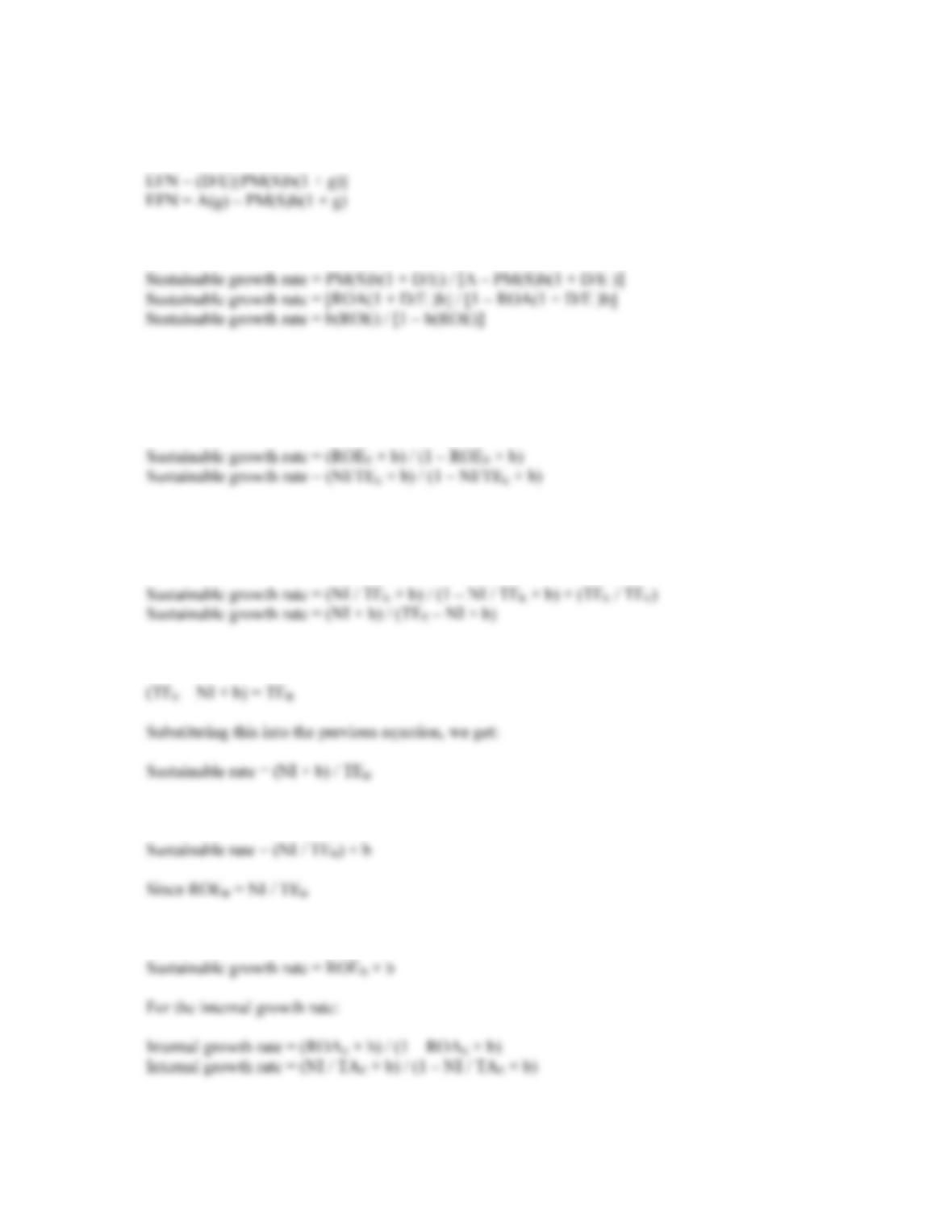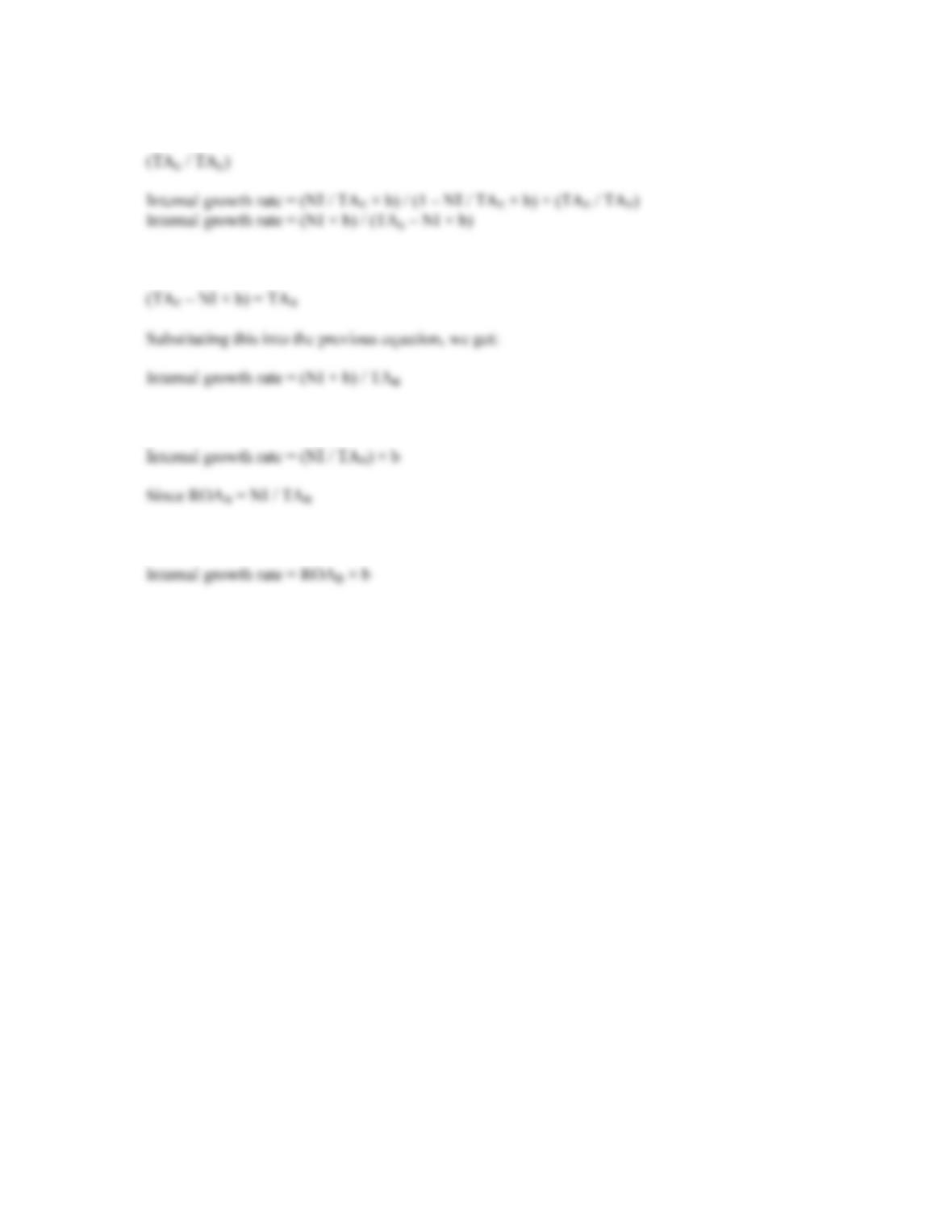Type
Quiz
Book Title
Fundamentals of Corporate Finance Standard Edition 9th Edition
ISBN 13
978-0073382395

### 978-0073382395 Chapter 4 Questions and Problems 32-33

April 3, 2019B-54 SOLUTIONS
This is a negative dividend payout ratio of 66 percent, which is impossible. The growth rate is not
consistent with the other constraints. The lowest possible payout rate is 0, which corresponds to
retention ratio of 1, or total earnings retention.
The maximum sustainable growth rate for this company is:
Maximum sustainable growth rate = (ROE × b) / [1 – (ROE × b)]
Maximum sustainable growth rate = [.0645(1)] / [1 – .0645(1)]
Maximum sustainable growth rate = .0690 or 6.90%
31. We know that EFN is:
The addition to retained earnings next year is the current net income times the retention ratio, times
one plus the growth rate, so:
Addition to retained earnings = (NI b)(1 + g)
And rearranging the profit margin to solve for net income, we get:
NI = PM(S)
Substituting the last three equations into the EFN equation we started with and rearranging, we get:
32. We start with the EFN equation we derived in Problem 31 and set it equal to zero:
Substituting the rearranged profit margin equation into the internal growth rate equation, we have:
Since:
We can substitute this into the internal growth rate equation and divide both the numerator and
denominator by A. This gives:CHAPTER 4 B-55
To derive the sustainable growth rate, we must realize that to maintain a constant D/E ratio with no
external equity financing, EFN must equal the addition to retained earnings times the D/E ratio:
Solving for g and then dividing numerator and denominator by A:
33. In the following derivations, the subscript “E” refers to end of period numbers, and the subscript “B”
refers to beginning of period numbers. TE is total equity and TA is total assets.
For the sustainable growth rate:
We multiply this equation by:
(TEE / TEE)
Recognize that the numerator is equal to beginning of period equity, that is:
Which is equivalent to:
The sustainable growth rate equation is:B-56 SOLUTIONS
We multiply this equation by:
Recognize that the numerator is equal to beginning of period assets, that is:
Which is equivalent to:
The internal growth rate equation is: